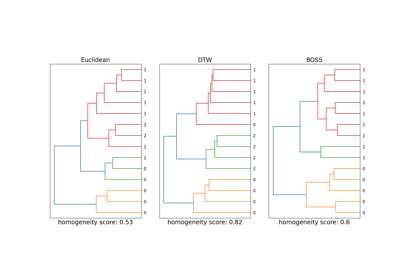# pyts.metrics.boss¶

pyts.metrics.boss(x, y)[source]

Return the BOSS distance between two arrays.

Parameters: x : array-like, shape = (n_timestamps,) First array. y : array-like, shape = (n_timestamps,) Second array. dist : float The BOSS distance between both arrays.

Notes

The BOSS metric is defined aswhereandare vectors of non-negative integers. The BOSS distance is not a distance metric as it neither satisfies the symmetry condition nor the triangle inequality.

References

  P. Schäfer, “The BOSS is concerned with time series classification in the presence of noise”. Data Mining and Knowledge Discovery, 29(6), 1505-1530 (2015).

Examples

>>> from pyts.metrics import boss
>>> x = [0, 5, 5, 3, 4, 5]
>>> y = [3, 0, 0, 0, 8, 0]
>>> boss(x, y)
10.0
>>> boss(y, x)
5.0


## Examples using pyts.metrics.boss¶Time Series Clustering with DTW and BOSS